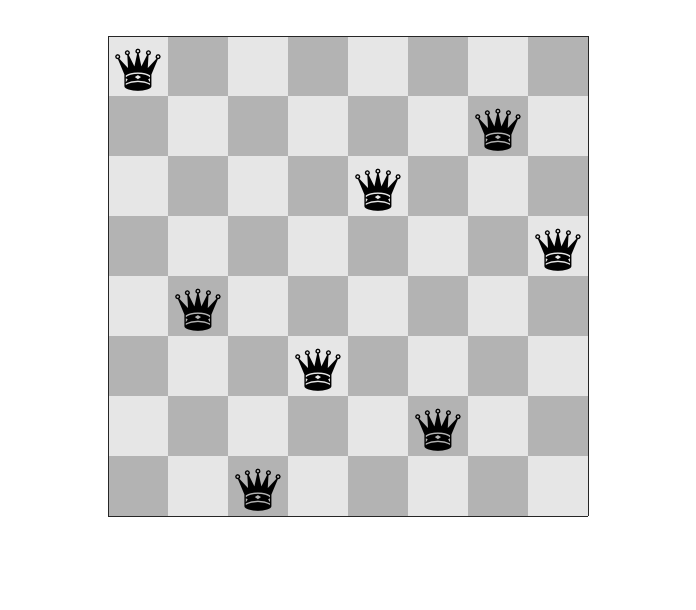# The Eight Queens Problem – Generating All Solutions

On April 20, I wrote about an algorithm for solving the Eight Queens Problem. In this problem, the task is to place eight queens on a chessboard so that none of the queens is attacking any other queen. Here is one solution.After I published that post, I became curious to see what others might have submitted about this problem on the File Exchange. A little searching brought me to this submission by uu tii. When I looked at the submission, I was surprised to how little code it contained. This is it:

function M = eightqueens()
N=8;
T=N-2;
rows=1:N;
cols=perms(rows);
S=size(cols,1);
M=zeros(N,N,S);
linearInd = sub2ind(size(M), repmat(rows',1,S), cols', repmat(1:S,N,1));
M(linearInd) = 1;
dv=arrayfun(@(k)max([arrayfun(@(x)sum(diag(M(:,:,k),x)),-T:T),arrayfun(@(x)sum(diag(rot90(M(:,:,k)),x)),-T:T)]),1:S);
M(:,:,dv>1)=[];

When I ran it, it returned an 8x8x92 array. The size caught my eye because there are exactly 92 solutions to the Eight Queens Problem (including rotations and flips).

M = eightqueens;
whos M

  Name      Size              Bytes  Class     Attributes

M         8x8x92            47104  double



Let's peek at one of the planes of M.

M(:,:,45)

ans =

0     0     0     0     1     0     0     0
1     0     0     0     0     0     0     0
0     0     0     0     0     0     0     1
0     0     0     1     0     0     0     0
0     1     0     0     0     0     0     0
0     0     0     0     0     0     1     0
0     0     1     0     0     0     0     0
0     0     0     0     0     1     0     0



Indeed, that is a solution. So, what's going on in this small chunk of code? Well, as I see it, this code has three conceptual chunks. The first chunk, consisting of eight lines, is the longest. Let's run it.

N=8;
T=N-2;
rows=1:N;
cols=perms(rows);
S=size(cols,1);
M=zeros(N,N,S);
linearInd = sub2ind(size(M), repmat(rows',1,S), cols', repmat(1:S,N,1));
M(linearInd) = 1;

whos M

  Name      Size                    Bytes  Class     Attributes

M         8x8x40320            20643840  double



This seems to be a collection of 40320 boards. There aren't that many solutions, so the collection must contain many boards that aren't valid solutions. For example:

M(:,:,45)

ans =

0     0     0     0     0     0     0     1
0     0     0     0     0     0     1     0
0     0     0     0     0     1     0     0
0     0     0     1     0     0     0     0
1     0     0     0     0     0     0     0
0     0     1     0     0     0     0     0
0     0     0     0     1     0     0     0
0     1     0     0     0     0     0     0



There are four queens lined up on the main antidiagonal, so this board is not a solution.

This set of boards has been constructed by making the observation that any solution must have exactly one queen on each row and exactly one queen on each column. The array M contains every board that has this property. All the computational magic here is in the line cols=perms(rows). The rest of this chunk is some indexing computations to initialize and fill in the array M with queens at the locations given by rows and cols.

The second chunk is this line:

dv=arrayfun(@(k)max([arrayfun(@(x)sum(diag(M(:,:,k),x)),-T:T),arrayfun(@(x)sum(diag(rot90(M(:,:,k)),x)),-T:T)]),1:S);


Wow, there's a lot going on there. I would summarize it this way: For every board k in M, sum every diagonal and every antidiagonal and take the maximum of those sums. The idea is that M was constructed so that every board has exactly one queen on each row and exactly one queen on each column, so you really only have to check the diagonals of a board to determine whether it is a solution. Let's look at dv for the 45th board, which I displayed above.

dv(45)

ans =

4



Remember my comment above that board 45 has 4 queens lined up on the main antidiagonal? That's why dv(45) is 4.

The last conceptual chunk is eliminate all the boards in the M that are not solutions. We do that by eliminating all boards for which dv > 1.

M(:,:,dv>1)=[];

whos M

  Name      Size              Bytes  Class     Attributes

M         8x8x92            47104  double



And there it is. That's a very clever use of perms and indexing and arrayfun to generate all possible solutions. Thanks, uu tii.

Published with MATLAB® R2017a

|

### Comments

To leave a comment, please click here to sign in to your MathWorks Account or create a new one.